Aashi Goyal — June 9, 2021

This article was published as a part of the Data Science Blogathon

## Introduction

While dealing with Statistical Moments (for a particular probability distribution either Continuous or Discrete) such as Mean, Variance, Skewness, etc, it becomes very important to have a good understanding of Moment Generating Functions (MGF).

So, In this article, we will be discussing the complete idea behind Moment Generating Functions including its applications with some examples.

1. What are Statistical Moments?

2. What is Moment Generating Function (MGF)?

3. Properties of MGF

4. Why do we need MGF?

5. Some Important Results of MGF

6. Applications of MGF

7. Problem Solving related to MGF

## What are Statistical Moments?

Let’s X be a random variable in which we are interested, then the moments are the expected values of X,

For Example, E(X), E(X²), E(X³), … etc.

The first moment is defined as E(X),

The second moment is defined as E(X²),

The third moment is defined as E(X³),

The n-th moment is defined as E(Xn).

In Statistics, we are pretty familiar with the first two moments:

• The Mean (μ) = E(X)
• The Variance (σ2) = E(X2) – (E(X))2 = E(X²) − μ²

These are the important characteristics for any general random variable X.

The mean denotes the average value and the variance represents how the data points are spread wrt mean in the distribution. But there must be other characteristics as well that also helps in defining the probability distributions.

For Example, In the third moment E(X3), Skewness tells about the asymmetry of distribution, and in the fourth moment E(X4), kurtosis tells about how heavy the tails of a distribution are.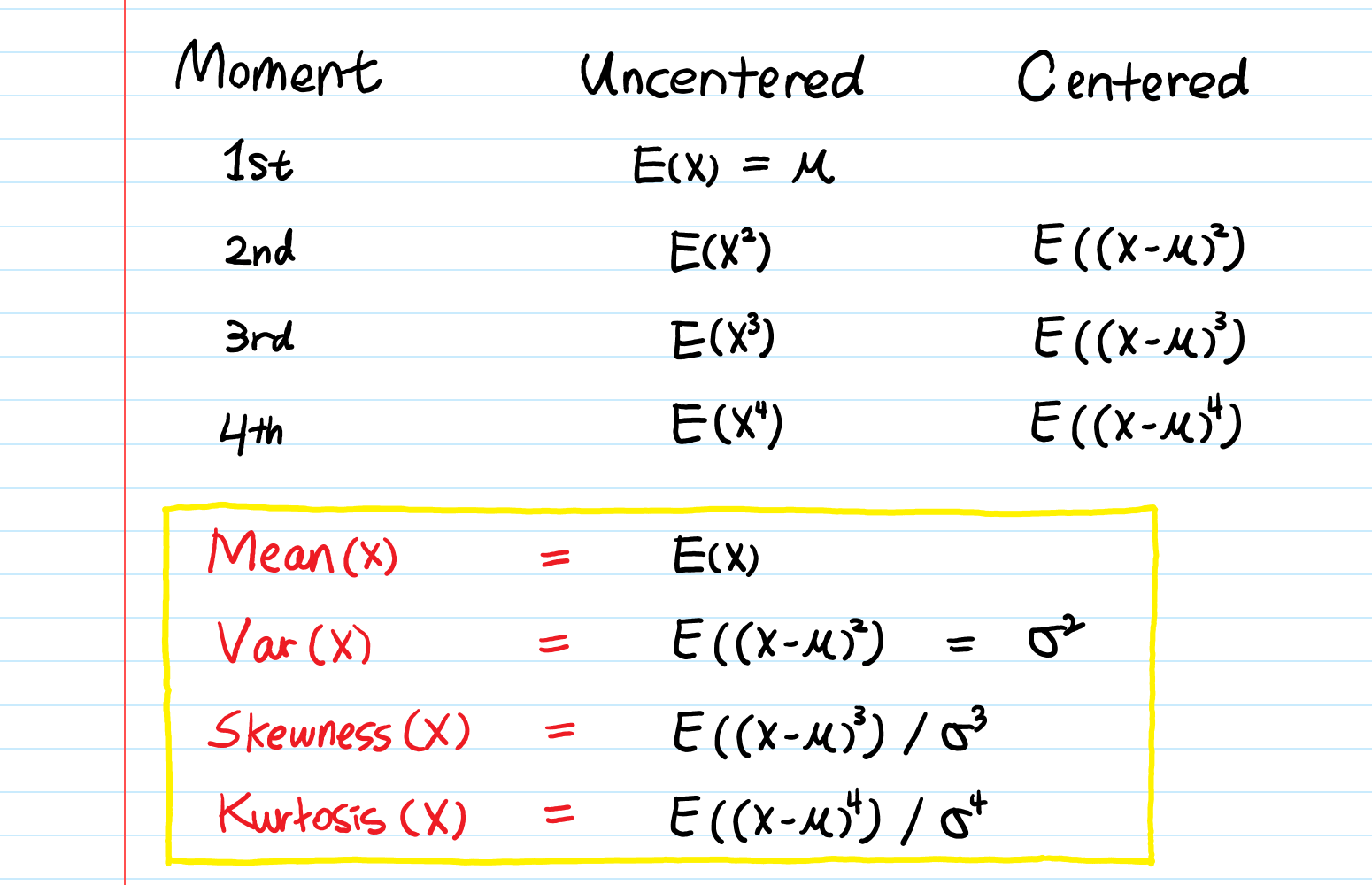## What is the Moment Generating Function?

The moment generating function (MGF) associated with a random variable X, is a function,

MX : R → [0,∞] defined by

MX(t) = E [ etX ]

The domain or region of convergence (ROC) of MX is the set DX = { t | MX(t) < ∞}.

In general, t can be a complex number, but since we did not define the expectations for complex-valued random variables, so we will restrict ourselves only to real-valued t. And the point to note that t= 0 is always a point in the ROC for any random variable since MX (0) = 1.

As its name implies, MGF is the function that generates the moments —

E(X), E(X²), E(X³), …, E(Xn).

Cases:

If X is discrete with probability mass function(pmf) pX (x), then

MX (t) = Σ etx pX (x)

If X is continuous with probability density function (pdf) fX (x), then

MX (t) = ∫ etx fX (x) dx

## Properties of Moment Generating Functions

1. Condition for a Valid MGF:

MX(0) = 1 i.e, Whenever you compute an MGF, plug in t = 0 and see if you get 1.

2. Moment Generating Property:

By looking at the definition of MGF, we might think that how we formulate it in the form of E(Xn) instead of E(etx).

So, to do this we take a derivative of MGF n times and plug t = 0 in. then, you will get E(Xn).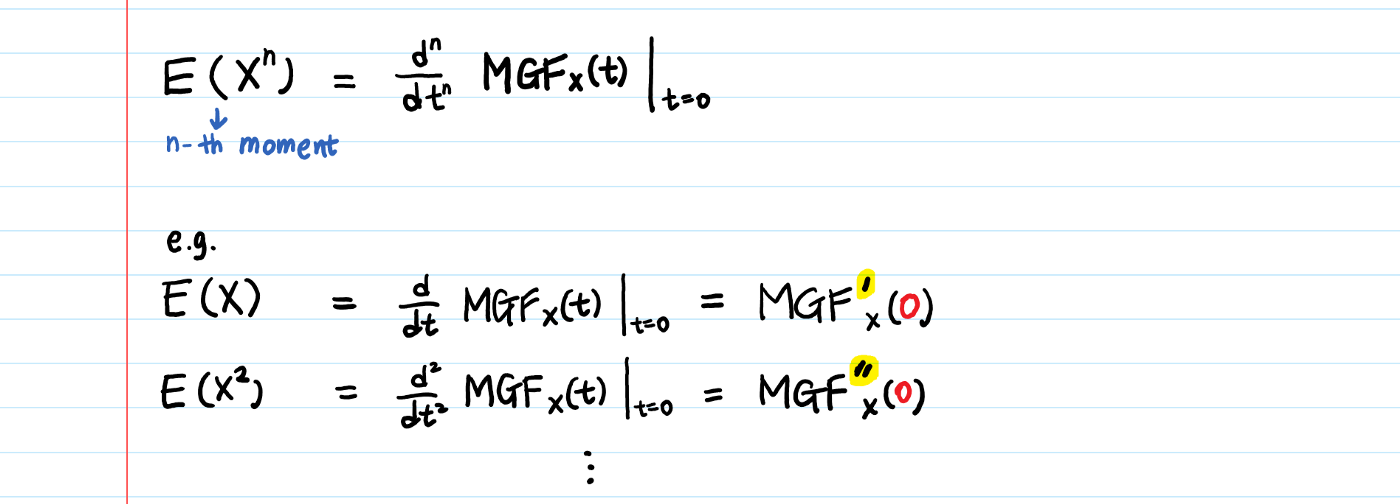Proof:

To prove the above property, we take the help of Taylor’s Series:

Step-1: Let’s see Taylor’s series Expansion of eX and then by using that expansion, we generate the expansion for etX which we will use in later steps.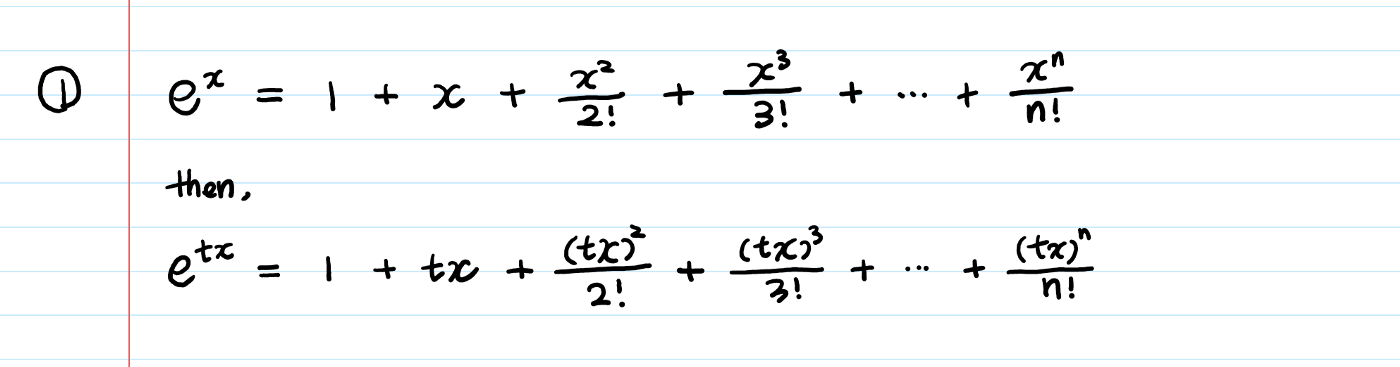Step-2: Take the expectation on both sides of the equation, we get: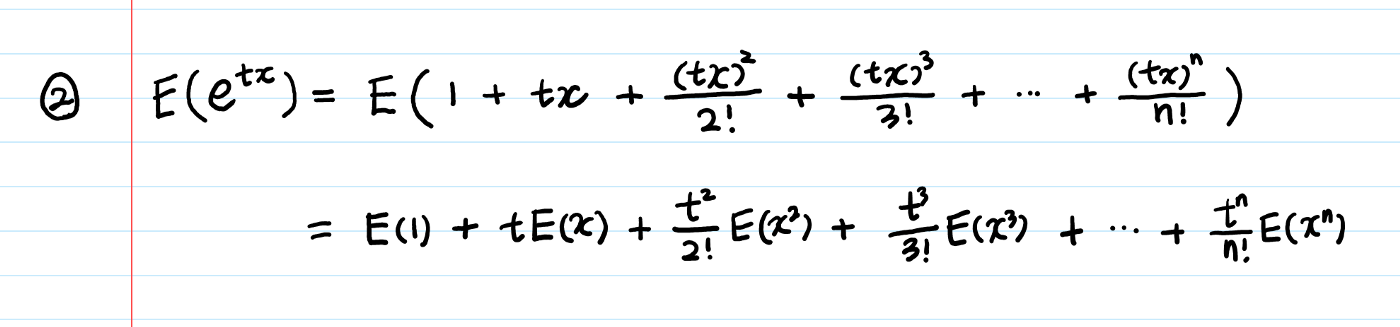Step-3: Now, take the derivative of the equation with respect to t and then we will reach our conclusion.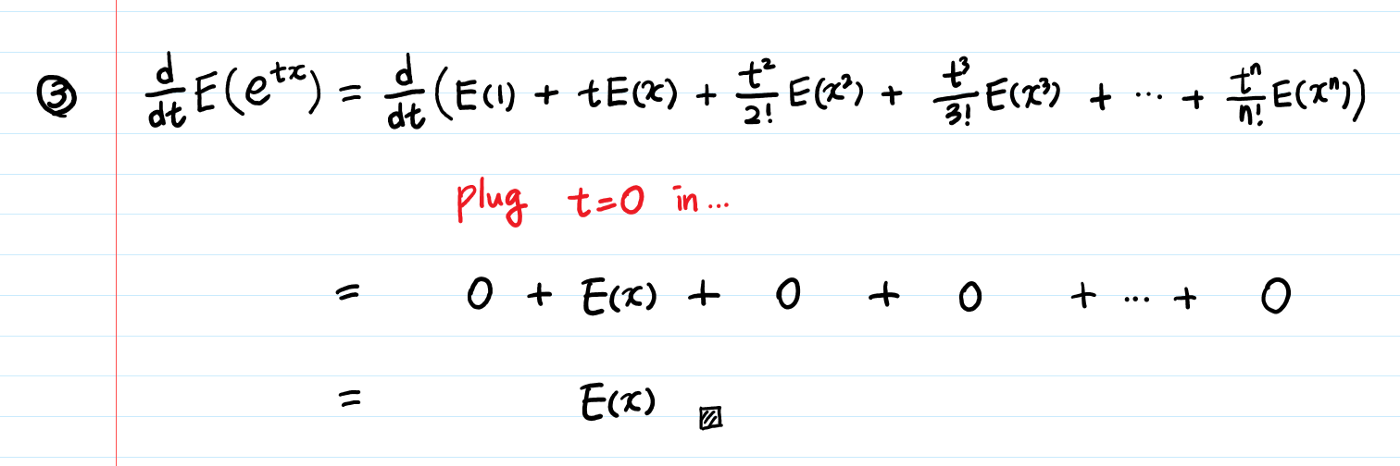In this step, we take the first derivative of the equation only but similarly, we can prove that:

• If you take another derivative on equation-3 (therefore total twice), you will get E(X²).
• If you take the third derivative, you will get E(X³), and so on.

Note:

When you try to deeply understand the concept behind the Moment Generating Function, we couldn’t understand the role of t in the function, since t seemed like some arbitrary variable that we are not interested in. However, as you see, t is considered as a helper variable.

So, to be able to use calculus (derivatives) and make the terms (that we are not interested in) zero, we introduced the variable t.

## Why do we need MGF?

We can calculate moments by using the definition of expected values but the question is that “Why do we need MGF exactly”?For convenience,

To calculate the moments easily, we have to use the MGF. But

“Why is the calculation of moments using MGF easier than by using the definition of expected values”?

Let’s understand this concept with the help of the given below example that will cause a spark of joy in you — the clearest example where MGF is easier:

Let’s try to find the MGF of the exponential distribution.

Step-1: Firstly, we will start our discussion by writing the PDF of Exponential Distribution.Step-2: With the help of pdf calculated in previous steps, now we determine the MGF of the exponential distribution.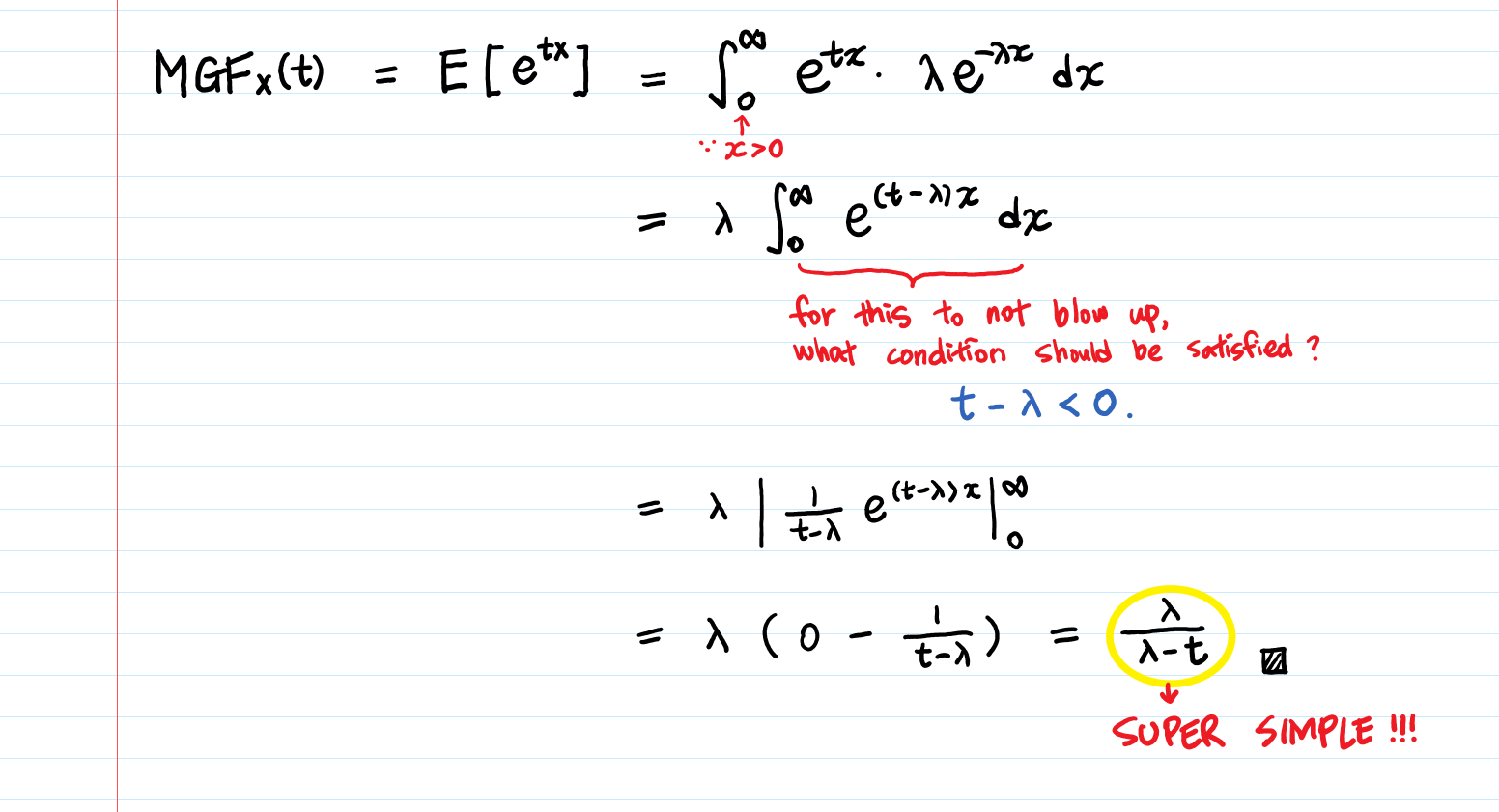Now, for MGF to exist, the expected value E(etx) should exist.

Therefore, `t – λ < 0` becomes an important condition to meet, because if this condition doesn’t hold, then the integral won’t converge. This is known as the Divergence Test.

Once you have to find the MGF of the exponential distribution to be λ/(λ-t), then calculating moments becomes just a matter of taking derivatives, which is easier than the integrals to calculate the expected value directly.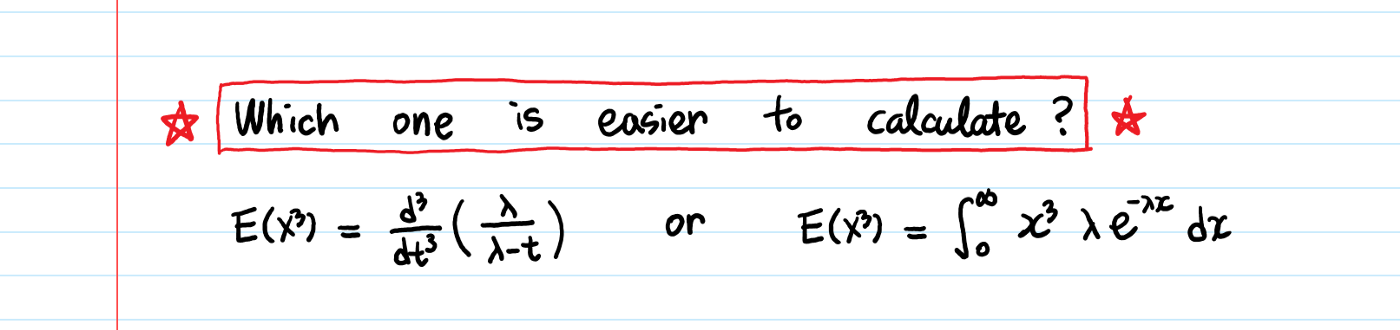Therefore, with the help of MGF, it is possible to find moments by taking derivatives rather than doing integrals! So, this makes our life easier when dealing with statistical moments.

## Important Results Related to MGF

Result-1: Sum of Independent Random Variables

Suppose X1,…, Xn are n independent random variables, and the random variable Y is defined by

Y = X1 + … + Xn.

Then, the moment generating function of random variable Y is given as,

MY(t)=MX1 (t)·…·MXn (t)

Result-2:

Suppose for two random variables X and Y we have MX(t) = MY (t) < ∞ for all t in an interval, then X and Y have the same distribution.

## Applications of MGF

1. Moments provide a way to specify a distribution:

We can completely specify the normal distribution by the first two moments, mean and variance. As we are going to know about multiple different moments of the distribution, then we will know more about that distribution.

For Example, If there is a person that you haven’t met, and you know about their height, weight, skin color, favorite hobby, etc., you still don’t necessarily fully know them but to getting more and more about them we can take the help of this.

2. Finding any n-th moment of a distribution:

We can get any n-th moment once you have MGF i.e, expected value exists. It encodes all the moments of a random variable into a single function from which we can be extracted again later.

3. Helps in determining Probability distribution uniquely:

Using MGF, we can uniquely determine a probability distribution. If two random variables have the same expression of MGF, then they must have the same probability distribution.

4. Risk Management in Finance:

In this domain, one of the important characteristics of distribution is how heavy its tails are.

For Example, If you know about the 2009 financial crisis, in which we were failing to address the possibility of rare events happening. So, risk managers try to understate the kurtosis, the fourth moment of many financial securities underlying the fund’s trading positions. So, sometimes seemingly random distributions with the help of hypothetically smooth curves of risk can have hidden bulges in them. So, to detect these bulges we can use the MGF.

## Problem Solving related to MGF

Numerical Example:

Suppose that Y is a random variable with MGF H(t). Further, suppose that X is also a random variable with MGF M(t) which is given by, M(t) = 1/3 (2e3t +1) H(t). Given that the mean of random variable Y is 10 and its variance is 12, then find the mean and variance of random variable X.

Solution:

Keep in mind all the results which we described above, we can say that

E(Y) = 10 ⇒ H'(0) =10,

E(Y2) – (E(Y))2 = 12 ⇒ E(Y2) – 100 = 12 ⇒ E(Y2) = 112 ⇒ H”(0) = 112

M'(t) = 2e3t H(t) + 1/3 ( 2e3t +1 )H'(t)

M”(t) = 6e3tH(t) + 4e3tH'(t) + 1/3 ( 2e3t +1 )H”(t)

Now, E(X) = M'(0) = 2H(0) + H'(0) = 2+10 =12

E(X2) = M”(0) = 6H(0) + 4H'(0) + H”(0) = 6 + 40 +112 = 158

Therefore, Var(X) = E(X2) – (E(X))2 = 158 -144 = 14

So, the mean and variance of Random variable X are 12 and 14 respectively.

This ends today’s discussion!

## Endnotes

I hope you enjoyed the article and increased your knowledge about Moment Generating Functions in Statistics.

Please feel free to contact me on Email

Something not mentioned or want to share your thoughts? Feel free to comment below And I’ll get back to you.

For the remaining articles, refer to the link.